## Cauchy Integral FormulaGiven a Contour Integral of the form(1)

define a pathas an infinitesimal Circle around the point(the dot in the above illustration). Define the pathas an arbitrary loop with a cut line (on which the forward and reverse contributions cancel each other out) so as to go around.

The total path is then(2)

so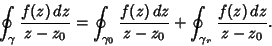(3)

From the Cauchy Integral Theorem, the Contour Integral along any path not enclosing a Pole is 0. Therefore, the first term in the above equation is 0 sincedoes not enclose the Pole, and we are left with(4)

Now, let, so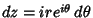. Then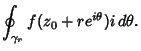(5)

But we are free to allow the radiusto shrink to 0, so(6)

and(7)

If multiple loops are made around the Pole, then equation (7) becomes(8)

whereis the Winding Number.

A similar formula holds for the derivatives of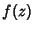,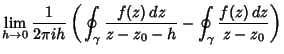(9)

Iterating again,(10)

Continuing the process and adding the Winding Number,(11)

Arfken, G. Cauchy's Integral Formula.'' §6.4 in Mathematical Methods for Physicists, 3rd ed. Orlando, FL: Academic Press, pp. 371-376, 1985.